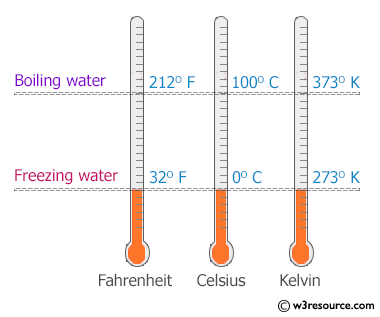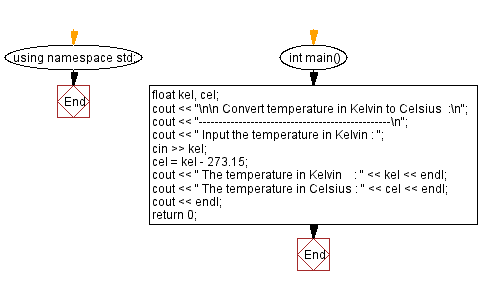﻿ C++ Exercises: Convert temperature in Kelvin to Celsius - w3resource

# C++ Exercises: Convert temperature in Kelvin to Celsius

## C++ Basic: Exercise-25 with Solution

Write a program in C++ to convert temperature in Kelvin to Celsius.

Visual Presentation:Sample Solution:

C++ Code :

``````#include <iostream> // Including the input-output stream header file

using namespace std; // Using the standard namespace

int main() // Start of the main function
{
float kel, cel; // Declaring floating-point variables for Kelvin and Celsius temperatures

cout << "\n\n Convert temperature in Kelvin to Celsius :\n"; // Outputting a message indicating temperature conversion
cout << "------------------------------------------------\n"; // Outputting a separator line

cout << " Input the temperature in Kelvin : "; // Prompting the user to input the temperature in Kelvin
cin >> kel; // Taking input for the temperature in Kelvin from the user

cel = kel - 273.15; // Converting Kelvin to Celsius using the conversion formula

cout << " The temperature in Kelvin    : " << kel << endl; // Displaying the input temperature in Kelvin
cout << " The temperature in Celsius : " << cel << endl; // Displaying the converted temperature in Celsius
cout << endl; // Outputting a blank line for better readability

return 0; // Returning 0 to indicate successful program execution
} // End of the main function
``````

Sample Output:

``` Convert temperature in Kelvin to Celsius  :
------------------------------------------------
Input the temperature in Kelvin : 300
The temperature in Kelvin    : 300
The temperature in Celsius : 26.85
```

Flowchart:C++ Code Editor:

What is the difficulty level of this exercise?

﻿